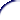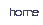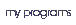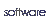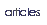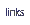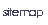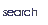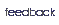## Programming in User RPL - Local variables

Let's see another simple program that does nothing more than calculate the hypotenuse of a triangle when the two catets are given:

`<< SQ SWAP SQ +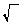>>`

In the above case, each of the two inputs is used only once, so stack-manipulation is not a real case. But in some cases, the inputs are used more than once, so keeping the stack organised is quite difficult and to get a specific value is a real problem. So, instead of using lots of commands just the get the value you need, why not store each of the values somewhere, and then recall them and if necessary change the value? It's a lot easier.

But where would you store the values. You could use variables, sure, but that's a problem. What if the user already has a variable with the name you want to use? And you must erase the variables used in the end of the program. But if the program terminates unexpectedly, because of an error, for example? The user's `VAR` menu would be full of unecessary stuff.

Because of this, the HP has a nice feature called local variables. They work like normal variables, but they don't appear on the `VAR` menu, are purged automatically, can't be acessed by the user and there is no problem in using a name that is already used by an user's variable.

The local variables are created with the `->` command. (actually it's the fancy arrow over the `0` key.) The use is very simple: anywhere you want, use `->` followed by as many names of variables you want, separeted by spaces. The values will be taken from the stack and stored in the variables.

Let's clarify this: suppose you have 1, 2 and 3 on the stack, on this order. If you use `-> a`, the local variable `a` will now contain the value 3. If you use `-> a b`, variable `a` will contain 2 and `b` will contain 3. And if you use `-> a b c`, `a` will contain 1, `b` 2 and `c` 3.

OK, but how do I use this in a program? Very simple: add the `->` command followed by the variable names and then either an algebraic expression that will be evaluated substituting any local variable names by their value; or a program that will be run, and where you can use any local variables as any local variable.

Clarifying: the above hypotenuse program can be written also as:

`<< -> a b '(a^2+b^2)' >>`   or
`<< -> a b << a SQ b SQ +>> >>`

But, in this case, the first on is the most effient. But in more complicated cases, local variables are much more efficient. At least, they make the programs easier to understand, because you can see the fourmulas, and not crypt stack manipulation commands like `SWAP 4 PICK OVER ROT ROT DUP`. However, you must know how the stack works, and the basic stack manipulation command before going further in this tutorial. Alas, by this time, you should already know them. If you don't, go back to the previous lesson and learn what they do.

#### Exercises

1. Given a, b, u, c, d and v on the stack, write a program to solve the systemTo make things easier: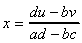2. Write a program, using local variables, to calculate the determinant of the matrix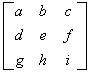, using:
1. Algebraic notation (ie, `-> a '4*a'`)
2. RPN notation (ie, `-> a << a 4 *`)
3. Write a program to calculate the inverse matrix of the matrix in the previous exercise.